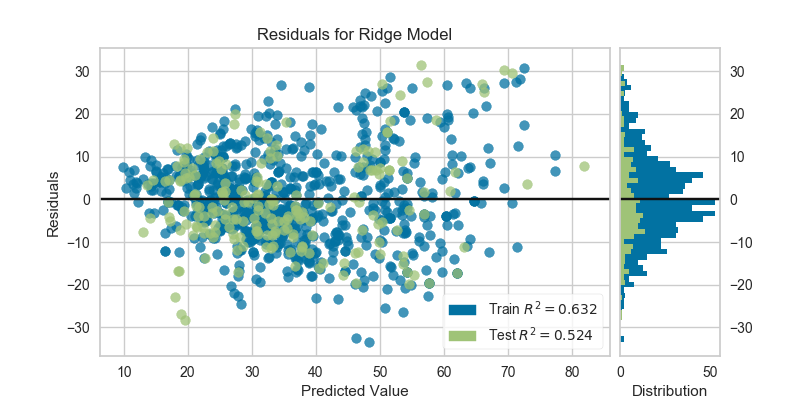# Residuals Plot¶

Residuals, in the context of regression models, are the difference between the observed value of the target variable (y) and the predicted value (ŷ), e.g. the error of the prediction. The residuals plot shows the difference between residuals on the vertical axis and the dependent variable on the horizontal axis, allowing you to detect regions within the target that may be susceptible to more or less error.

from sklearn.model_selection import train_test_split

# Load the data

# Identify the feature and target columns
feature_names = [
'cement', 'slag', 'ash', 'water', 'splast', 'coarse', 'fine', 'age'
]
target_name = 'strength'

# Separate the instance data from the target data
X = df[feature_names]
y = df[target_name]

# Create the train and test data
X_train, X_test, y_train, y_test = train_test_split(X, y, test_size=0.2)

from sklearn.linear_model import Ridge
from yellowbrick.regressor import ResidualsPlot

# Instantiate the linear model and visualizer
ridge = Ridge()
visualizer = ResidualsPlot(ridge)

visualizer.fit(X_train, y_train)  # Fit the training data to the model
visualizer.score(X_test, y_test)  # Evaluate the model on the test data
visualizer.poof()                 # Draw/show/poof the dataA common use of the residuals plot is to analyze the variance of the error of the regressor. If the points are randomly dispersed around the horizontal axis, a linear regression model is usually appropriate for the data; otherwise, a non-linear model is more appropriate. In the case above, we see a fairly random, uniform distribution of the residuals against the target in two dimensions. This seems to indicate that our linear model is performing well. We can also see from the histogram that our error is normally distributed around zero, which also generally indicates a well fitted model.

Note that if the histogram is not desired, it can be turned off with the hist=False flag:

visualizer = ResidualsPlot(ridge, hist=False)
visualizer.fit(X_train, y_train)
visualizer.score(X_test, y_test)
visualizer.poof()Warning

The histogram on the residuals plot requires matplotlib 2.0.2 or greater. If you are using an earlier version of matplotlib, simply set the hist=False flag so that the histogram is not drawn.

## API Reference¶

Regressor visualizers that score residuals: prediction vs. actual data.

class yellowbrick.regressor.residuals.ResidualsPlot(model, ax=None, hist=True, train_color='b', test_color='g', line_color='#111111', alpha=0.75, **kwargs)[source]

Bases: yellowbrick.regressor.base.RegressionScoreVisualizer

A residual plot shows the residuals on the vertical axis and the independent variable on the horizontal axis.

If the points are randomly dispersed around the horizontal axis, a linear regression model is appropriate for the data; otherwise, a non-linear model is more appropriate.

Parameters: model : a Scikit-Learn regressor Should be an instance of a regressor, otherwise will raise a YellowbrickTypeError exception on instantiation. ax : matplotlib Axes, default: None The axes to plot the figure on. If None is passed in the current axes will be used (or generated if required). hist : {True, False, None, ‘density’, ‘frequency’}, default: True Draw a histogram showing the distribution of the residuals on the right side of the figure. Requires Matplotlib >= 2.0.2. If set to ‘density’, the probability density function will be plotted. If set to True or ‘frequency’ then the frequency will be plotted. train_color : color, default: ‘b’ Residuals for training data are ploted with this color but also given an opacity of 0.5 to ensure that the test data residuals are more visible. Can be any matplotlib color. test_color : color, default: ‘g’ Residuals for test data are plotted with this color. In order to create generalizable models, reserved test data residuals are of the most analytical interest, so these points are highlighted by having full opacity. Can be any matplotlib color. line_color : color, default: dark grey Defines the color of the zero error line, can be any matplotlib color. alpha : float, default: 0.75 Specify a transparency where 1 is completely opaque and 0 is completely transparent. This property makes densely clustered points more visible. kwargs : dict Keyword arguments that are passed to the base class and may influence the visualization as defined in other Visualizers.

Notes

ResidualsPlot is a ScoreVisualizer, meaning that it wraps a model and its primary entry point is the score() method.

The residuals histogram feature requires matplotlib 2.0.2 or greater.

Examples

>>> from yellowbrick.regressor import ResidualsPlot
>>> from sklearn.linear_model import Ridge
>>> model = ResidualsPlot(Ridge())
>>> model.fit(X_train, y_train)
>>> model.score(X_test, y_test)
>>> model.poof()

draw(y_pred, residuals, train=False, **kwargs)[source]

Draw the residuals against the predicted value for the specified split. It is best to draw the training split first, then the test split so that the test split (usually smaller) is above the training split; particularly if the histogram is turned on.

Parameters: y_pred : ndarray or Series of length n An array or series of predicted target values residuals : ndarray or Series of length n An array or series of the difference between the predicted and the target values train : boolean, default: False If False, draw assumes that the residual points being plotted are from the test data; if True, draw assumes the residuals are the train data. Returns —— ax : the axis with the plotted figure
finalize(**kwargs)[source]

Finalize executes any subclass-specific axes finalization steps. The user calls poof and poof calls finalize.

Parameters: kwargs: generic keyword arguments.
fit(X, y, **kwargs)[source]
Parameters: X : ndarray or DataFrame of shape n x m A matrix of n instances with m features y : ndarray or Series of length n An array or series of target values kwargs: keyword arguments passed to Scikit-Learn API. self : visualizer instance
hax

Returns the histogram axes, creating it only on demand.

score(X, y=None, train=False, **kwargs)[source]

Generates predicted target values using the Scikit-Learn estimator.

Parameters: X : array-like X (also X_test) are the dependent variables of test set to predict y : array-like y (also y_test) is the independent actual variables to score against train : boolean If False, score assumes that the residual points being plotted are from the test data; if True, score assumes the residuals are the train data. Returns —— score : float The score of the underlying estimator, usually the R-squared score for regression estimators.